Related Articles

# Boyer Moore Algorithm | Good Suffix heuristic

• Difficulty Level : Expert
• Last Updated : 31 Oct, 2019

We have already discussed Bad character heuristic variation of Boyer Moore algorithm. In this article we will discuss Good Suffix heuristic for pattern searching. Just like bad character heuristic, a preprocessing table is generated for good suffix heuristic.

Good Suffix Heuristic

Let t be substring of text T which is matched with substring of pattern P. Now we shift pattern until :
1) Another occurrence of t in P matched with t in T.
2) A prefix of P, which matches with suffix of t
3) P moves past t

Case 1: Another occurrence of t in P matched with t in T
Pattern P might contain few more occurrences of t. In such case, we will try to shift the pattern to align that occurrence with t in text T. For example-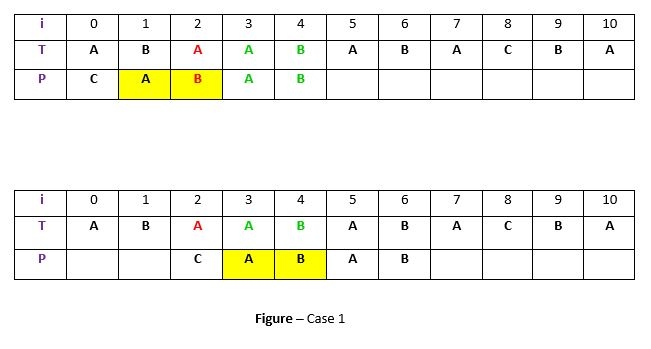Explanation: In the above example, we have got a substring t of text T matched with pattern P (in green) before mismatch at index 2. Now we will search for occurrence of t (“AB”) in P. We have found an occurrence starting at position 1 (in yellow background) so we will right shift the pattern 2 times to align t in P with t in T. This is weak rule of original Boyer Moore and not much effective, we will discuss a Strong Good Suffix rule shortly.

Case 2: A prefix of P, which matches with suffix of t in T
It is not always likely that we will find the occurrence of t in P. Sometimes there is no occurrence at all, in such cases sometimes we can search for some suffix of t matching with some prefix of P and try to align them by shifting P. For example –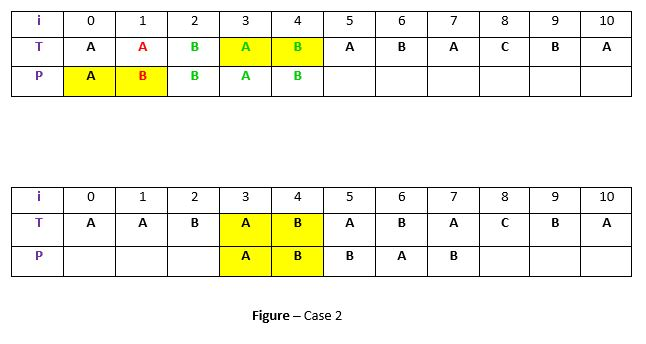Explanation: In above example, we have got t (“BAB”) matched with P (in green) at index 2-4 before mismatch . But because there exists no occurrence of t in P we will search for some prefix of P which matches with some suffix of t. We have found prefix “AB” (in the yellow background) starting at index 0 which matches not with whole t but the suffix of t “AB” starting at index 3. So now we will shift pattern 3 times to align prefix with the suffix.

Case 3: P moves past t
If the above two cases are not satisfied, we will shift the pattern past the t. For example –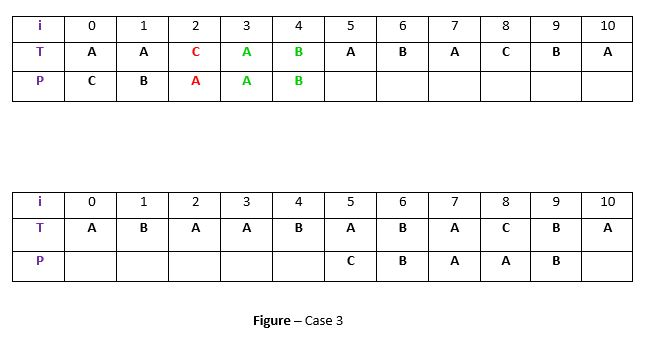Explanation: If above example, there exist no occurrence of t (“AB”) in P and also there is no prefix in P which matches with the suffix of t. So, in that case, we can never find any perfect match before index 4, so we will shift the P past the t ie. to index 5.

Strong Good suffix Heuristic

Suppose substring q = P[i to n] got matched with t in T and c = P[i-1] is the mismatching character. Now unlike case 1 we will search for t in P which is not preceded by character c. The closest such occurrence is then aligned with t in T by shifting pattern P. For example –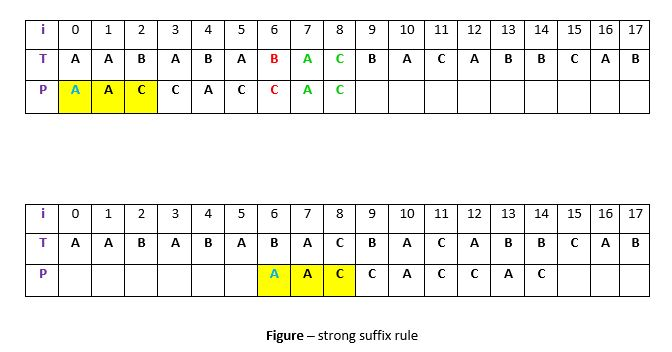Explanation: In above example, q = P[7 to 8] got matched with t in T. The mismatching character c is “C” at position P. Now if we start searching t in P we will get the first occurrence of t starting at position 4. But this occurrence is preceded by “C” which is equal to c, so we will skip this and carry on searching. At position 1 we got another occurrence of t (in the yellow background). This occurrence is preceded by “A” (in blue) which is not equivalent to c. So we will shift pattern P 6 times to align this occurrence with t in T.We are doing this because we already know that character c = “C” causes the mismatch. So any occurrence of t preceded by c will again cause mismatch when aligned with t, so that’s why it is better to skip this.

Preprocessing for Good suffix heuristic

As a part of preprocessing, an array shift is created. Each entry shift[i] contain the distance pattern will shift if mismatch occur at position i-1. That is, the suffix of pattern starting at position i is matched and a mismatch occur at position i-1. Preprocessing is done separately for strong good suffix and case 2 discussed above.

1) Preprocessing for Strong Good Suffix
Before discussing preprocessing, let us first discuss the idea of border. A border is a substring which is both proper suffix and proper prefix. For example, in string “ccacc”, “c” is a border, “cc” is a border because it appears in both end of string but “cca” is not a border.

As a part of preprocessing an array bpos (border position) is calculated. Each entry bpos[i] contains the starting index of border for suffix starting at index i in given pattern P.
The suffix φ beginning at position m has no border, so bpos[m] is set to m+1 where m is the length of the pattern.
The shift position is obtained by the borders which cannot be extended to the left. Following is the code for preprocessing –

```void preprocess_strong_suffix(int *shift, int *bpos,
char *pat, int m)
{
int i = m, j = m+1;
bpos[i] = j;
while(i > 0)
{
while(j <= m && pat[i-1] != pat[j-1])
{
if (shift[j] == 0)
shift[j] = j-i;
j = bpos[j];
}
i--; j--;
bpos[i] = j;
}
}
```

Explanation: Consider pattern P = “ABBABAB”, m = 7.

• The widest border of suffix “AB” beginning at position i = 5 is φ(nothing) starting at position 7 so bpos = 7.
• At position i = 2 the suffix is “BABAB”. The widest border for this suffix is “BAB” starting at position 4, so j = bpos = 4.

We can understand bpos[i] = j using following example –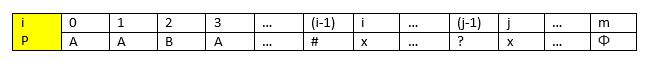If character # Which is at position i-1 is equivalent to character ? at position j-1, we know that border will be ? + border of suffix at position i which is starting at position j which is equivalent to saying that border of suffix at i-1 begin at j-1 or bpos[ i-1 ] = j-1 or in the code –

```i--;
j--;
bpos[ i ] = j
```

But if character # at position i-1 do not match with character ? at position j-1 then we continue our search to the right. Now we know that –

1. Border width will be smaller than the border starting at position j ie. smaller than x…φ
2. Border has to begin with # and end with φ or could be empty (no border exist).

With above two facts we will continue our search in sub string x…φ from position j to m. The next border should be at j = bpos[j]. After updating j, we again compare character at position j-1 (?) with # and if they are equal then we got our border otherwise we continue our search to right until j>m. This process is shown by code –

```while(j <= m && pat[i-1] != pat[j-1])
{
j = bpos[j];
}
i--; j--;
bpos[i]=j;
```

In above code look at these conditions –

```pat[i-1] != pat[j-1]
```

This is the condition which we discussed in case 2. When the character preceding the occurrence of t in pattern P is different than mismatching character in P, we stop skipping the occurrences and shift the pattern. So here P[i] == P[j] but P[i-1] != p[j-1] so we shift pattern from i to j. So shift[j] = j-i is recorder for j. So whenever any mismatch occur at position j we will shift the pattern shift[j+1] positions to the right.
In above code the following condition is very important –

```if (shift[j] == 0 )
```

This condition prevent modification of shift[j] value from suffix having same border. For example, Consider pattern P = “addbddcdd”, when we calculate bpos[ i-1 ] for i = 4 then j = 7 in this case. we will be eventually setting value of shift[ 7 ] = 3. Now if we calculate bpos[ i-1 ] for i = 1 then j = 7 and we will be setting value shift[ 7 ] = 6 again if there is no test shift[ j ] == 0. This mean if we have a mismatch at position 6 we will shift pattern P 3 positions to right not 6 position.

2) Preprocessing for Case 2
In the preprocessing for case 2, for each suffix the widest border of the whole pattern that is contained in that suffix is determined.
The starting position of the widest border of the pattern at all is stored in bpos
In the following preprocessing algorithm, this value bpos is stored initially in all free entries of array shift. But when the suffix of the pattern becomes shorter than bpos, the algorithm continues with the next-wider border of the pattern, i.e. with bpos[j].

Following is the implementation of the search algorithm –

## C++

 `/* C program for Boyer Moore Algorithm with ``   ``Good Suffix heuristic to find pattern in``   ``given text string */`` ` `#include ``#include `` ` `// preprocessing for strong good suffix rule``void` `preprocess_strong_suffix(``int` `*shift, ``int` `*bpos,``                                ``char` `*pat, ``int` `m)``{``    ``// m is the length of pattern ``    ``int` `i=m, j=m+1;``    ``bpos[i]=j;`` ` `    ``while``(i>0)``    ``{``        ``/*if character at position i-1 is not equivalent to``          ``character at j-1, then continue searching to right``          ``of the pattern for border */``        ``while``(j<=m && pat[i-1] != pat[j-1])``        ``{``            ``/* the character preceding the occurrence of t in ``               ``pattern P is different than the mismatching character in P, ``               ``we stop skipping the occurrences and shift the pattern``               ``from i to j */``            ``if` `(shift[j]==0)``                ``shift[j] = j-i;`` ` `            ``//Update the position of next border ``            ``j = bpos[j];``        ``}``        ``/* p[i-1] matched with p[j-1], border is found.``           ``store the  beginning position of border */``        ``i--;j--;``        ``bpos[i] = j; ``    ``}``}`` ` `//Preprocessing for case 2``void` `preprocess_case2(``int` `*shift, ``int` `*bpos,``                      ``char` `*pat, ``int` `m)``{``    ``int` `i, j;``    ``j = bpos;``    ``for``(i=0; i<=m; i++)``    ``{``        ``/* set the border position of the first character of the pattern``           ``to all indices in array shift having shift[i] = 0 */` `        ``if``(shift[i]==0)``            ``shift[i] = j;`` ` `        ``/* suffix becomes shorter than bpos, use the position of ``           ``next widest border as value of j */``        ``if` `(i==j)``            ``j = bpos[j];``    ``}``}`` ` `/*Search for a pattern in given text using``  ``Boyer Moore algorithm with Good suffix rule */``void` `search(``char` `*text, ``char` `*pat)``{``    ``// s is shift of the pattern with respect to text``    ``int` `s=0, j;``    ``int` `m = ``strlen``(pat);``    ``int` `n = ``strlen``(text);`` ` `    ``int` `bpos[m+1], shift[m+1];`` ` `    ``//initialize all occurrence of shift to 0``    ``for``(``int` `i=0;i= 0 && pat[j] == text[s+j])``            ``j--;`` ` `        ``/* If the pattern is present at the current shift, then index j``             ``will become -1 after the above loop */``        ``if` `(j<0)``        ``{``            ``printf``(``"pattern occurs at shift = %d\n"``, s);``            ``s += shift;``        ``}``        ``else``            ``/*pat[i] != pat[s+j] so shift the pattern``              ``shift[j+1] times  */``            ``s += shift[j+1];``    ``}`` ` `}`` ` `//Driver ``int` `main()``{``    ``char` `text[] = ``"ABAAAABAACD"``;``    ``char` `pat[] = ``"ABA"``;``    ``search(text, pat);``    ``return` `0;``}`

## Java

 `/* Java program for Boyer Moore Algorithm with ``Good Suffix heuristic to find pattern in``given text string */``class` `GFG ``{`` ` `// preprocessing for strong good suffix rule``static` `void` `preprocess_strong_suffix(``int` `[]shift, ``int` `[]bpos,``                                      ``char` `[]pat, ``int` `m)``{``    ``// m is the length of pattern ``    ``int` `i = m, j = m + ``1``;``    ``bpos[i] = j;`` ` `    ``while``(i > ``0``)``    ``{``        ``/*if character at position i-1 is not ``        ``equivalent to character at j-1, then ``        ``continue searching to right of the``        ``pattern for border */``        ``while``(j <= m && pat[i - ``1``] != pat[j - ``1``])``        ``{``            ``/* the character preceding the occurrence of t ``            ``in pattern P is different than the mismatching ``            ``character in P, we stop skipping the occurrences ``            ``and shift the pattern from i to j */``            ``if` `(shift[j] == ``0``)``                ``shift[j] = j - i;`` ` `            ``//Update the position of next border ``            ``j = bpos[j];``        ``}``        ``/* p[i-1] matched with p[j-1], border is found.``        ``store the beginning position of border */``        ``i--; j--;``        ``bpos[i] = j; ``    ``}``}`` ` `//Preprocessing for case 2``static` `void` `preprocess_case2(``int` `[]shift, ``int` `[]bpos,``                              ``char` `[]pat, ``int` `m)``{``    ``int` `i, j;``    ``j = bpos[``0``];``    ``for``(i = ``0``; i <= m; i++)``    ``{``        ``/* set the border position of the first character ``        ``of the pattern to all indices in array shift``        ``having shift[i] = 0 */``        ``if``(shift[i] == ``0``)``            ``shift[i] = j;`` ` `        ``/* suffix becomes shorter than bpos, ``        ``use the position of next widest border``        ``as value of j */``        ``if` `(i == j)``            ``j = bpos[j];``    ``}``}`` ` `/*Search for a pattern in given text using``Boyer Moore algorithm with Good suffix rule */``static` `void` `search(``char` `[]text, ``char` `[]pat)``{``    ``// s is shift of the pattern ``    ``// with respect to text``    ``int` `s = ``0``, j;``    ``int` `m = pat.length;``    ``int` `n = text.length;`` ` `    ``int` `[]bpos = ``new` `int``[m + ``1``];``    ``int` `[]shift = ``new` `int``[m + ``1``];`` ` `    ``//initialize all occurrence of shift to 0``    ``for``(``int` `i = ``0``; i < m + ``1``; i++) ``        ``shift[i] = ``0``;`` ` `    ``//do preprocessing``    ``preprocess_strong_suffix(shift, bpos, pat, m);``    ``preprocess_case2(shift, bpos, pat, m);`` ` `    ``while``(s <= n - m)``    ``{``        ``j = m - ``1``;`` ` `        ``/* Keep reducing index j of pattern while ``        ``characters of pattern and text are matching ``        ``at this shift s*/``        ``while``(j >= ``0` `&& pat[j] == text[s+j])``            ``j--;`` ` `        ``/* If the pattern is present at the current shift, ``        ``then index j will become -1 after the above loop */``        ``if` `(j < ``0``)``        ``{``            ``System.out.printf(``"pattern occurs at shift = %d\n"``, s);``            ``s += shift[``0``];``        ``}``        ``else``         ` `            ``/*pat[i] != pat[s+j] so shift the pattern``            ``shift[j+1] times */``            ``s += shift[j + ``1``];``    ``}`` ` `}`` ` `// Driver Code``public` `static` `void` `main(String[] args) ``{``    ``char` `[]text = ``"ABAAAABAACD"``.toCharArray();``    ``char` `[]pat = ``"ABA"``.toCharArray();``    ``search(text, pat);``}``} `` ` `// This code is contributed by 29AjayKumar`

## Python3

 `# Python3 program for Boyer Moore Algorithm with ``# Good Suffix heuristic to find pattern in ``# given text string`` ` `# preprocessing for strong good suffix rule``def` `preprocess_strong_suffix(shift, bpos, pat, m):`` ` `    ``# m is the length of pattern``    ``i ``=` `m``    ``j ``=` `m ``+` `1``    ``bpos[i] ``=` `j`` ` `    ``while` `i > ``0``:``         ` `        ``'''if character at position i-1 is ``        ``not equivalent to character at j-1, ``        ``then continue searching to right ``        ``of the pattern for border '''``        ``while` `j <``=` `m ``and` `pat[i ``-` `1``] !``=` `pat[j ``-` `1``]:``             ` `            ``''' the character preceding the occurrence ``            ``of t in pattern P is different than the ``            ``mismatching character in P, we stop skipping``            ``the occurrences and shift the pattern ``            ``from i to j '''``            ``if` `shift[j] ``=``=` `0``:``                ``shift[j] ``=` `j ``-` `i`` ` `            ``# Update the position of next border``            ``j ``=` `bpos[j]``             ` `        ``''' p[i-1] matched with p[j-1], border is found. ``        ``store the beginning position of border '''``        ``i ``-``=` `1``        ``j ``-``=` `1``        ``bpos[i] ``=` `j`` ` `# Preprocessing for case 2``def` `preprocess_case2(shift, bpos, pat, m):``    ``j ``=` `bpos[``0``]``    ``for` `i ``in` `range``(m ``+` `1``):``         ` `        ``''' set the border position of the first character ``        ``of the pattern to all indices in array shift``        ``having shift[i] = 0 '''``        ``if` `shift[i] ``=``=` `0``:``            ``shift[i] ``=` `j``             ` `        ``''' suffix becomes shorter than bpos, ``        ``use the position of next widest border``        ``as value of j '''``        ``if` `i ``=``=` `j:``            ``j ``=` `bpos[j]`` ` `'''Search for a pattern in given text using ``Boyer Moore algorithm with Good suffix rule '''``def` `search(text, pat):`` ` `    ``# s is shift of the pattern with respect to text``    ``s ``=` `0``    ``m ``=` `len``(pat)``    ``n ``=` `len``(text)`` ` `    ``bpos ``=` `[``0``] ``*` `(m ``+` `1``)`` ` `    ``# initialize all occurrence of shift to 0``    ``shift ``=` `[``0``] ``*` `(m ``+` `1``)`` ` `    ``# do preprocessing``    ``preprocess_strong_suffix(shift, bpos, pat, m)``    ``preprocess_case2(shift, bpos, pat, m)`` ` `    ``while` `s <``=` `n ``-` `m:``        ``j ``=` `m ``-` `1``         ` `        ``''' Keep reducing index j of pattern while characters of ``            ``pattern and text are matching at this shift s'''``        ``while` `j >``=` `0` `and` `pat[j] ``=``=` `text[s ``+` `j]:``            ``j ``-``=` `1``             ` `        ``''' If the pattern is present at the current shift, ``            ``then index j will become -1 after the above loop '''``        ``if` `j < ``0``:``            ``print``(``"pattern occurs at shift = %d"` `%` `s)``            ``s ``+``=` `shift[``0``]``        ``else``:``             ` `            ``'''pat[i] != pat[s+j] so shift the pattern ``            ``shift[j+1] times '''``            ``s ``+``=` `shift[j ``+` `1``]`` ` `# Driver Code``if` `__name__ ``=``=` `"__main__"``:``    ``text ``=` `"ABAAAABAACD"``    ``pat ``=` `"ABA"``    ``search(text, pat)`` ` `# This code is contributed by``# sanjeev2552`

## C#

 `/* C# program for Boyer Moore Algorithm with ``Good Suffix heuristic to find pattern in``given text string */``using` `System;`` ` `class` `GFG ``{`` ` `// preprocessing for strong good suffix rule``static` `void` `preprocess_strong_suffix(``int` `[]shift, ``                                     ``int` `[]bpos,``                                     ``char` `[]pat, ``int` `m)``{``    ``// m is the length of pattern ``    ``int` `i = m, j = m + 1;``    ``bpos[i] = j;`` ` `    ``while``(i > 0)``    ``{``        ``/*if character at position i-1 is not ``        ``equivalent to character at j-1, then ``        ``continue searching to right of the``        ``pattern for border */``        ``while``(j <= m && pat[i - 1] != pat[j - 1])``        ``{``            ``/* the character preceding the occurrence of t ``            ``in pattern P is different than the mismatching ``            ``character in P, we stop skipping the occurrences ``            ``and shift the pattern from i to j */``            ``if` `(shift[j] == 0)``                ``shift[j] = j - i;`` ` `            ``//Update the position of next border ``            ``j = bpos[j];``        ``}``        ``/* p[i-1] matched with p[j-1], border is found.``        ``store the beginning position of border */``        ``i--; j--;``        ``bpos[i] = j; ``    ``}``}`` ` `//Preprocessing for case 2``static` `void` `preprocess_case2(``int` `[]shift, ``int` `[]bpos,``                             ``char` `[]pat, ``int` `m)``{``    ``int` `i, j;``    ``j = bpos;``    ``for``(i = 0; i <= m; i++)``    ``{``        ``/* set the border position of the first character ``        ``of the pattern to all indices in array shift``        ``having shift[i] = 0 */``        ``if``(shift[i] == 0)``            ``shift[i] = j;`` ` `        ``/* suffix becomes shorter than bpos, ``        ``use the position of next widest border``        ``as value of j */``        ``if` `(i == j)``            ``j = bpos[j];``    ``}``}`` ` `/*Search for a pattern in given text using``Boyer Moore algorithm with Good suffix rule */``static` `void` `search(``char` `[]text, ``char` `[]pat)``{``    ``// s is shift of the pattern ``    ``// with respect to text``    ``int` `s = 0, j;``    ``int` `m = pat.Length;``    ``int` `n = text.Length;`` ` `    ``int` `[]bpos = ``new` `int``[m + 1];``    ``int` `[]shift = ``new` `int``[m + 1];`` ` `    ``// initialize all occurrence of shift to 0``    ``for``(``int` `i = 0; i < m + 1; i++) ``        ``shift[i] = 0;`` ` `    ``// do preprocessing``    ``preprocess_strong_suffix(shift, bpos, pat, m);``    ``preprocess_case2(shift, bpos, pat, m);`` ` `    ``while``(s <= n - m)``    ``{``        ``j = m - 1;`` ` `        ``/* Keep reducing index j of pattern while ``        ``characters of pattern and text are matching ``        ``at this shift s*/``        ``while``(j >= 0 && pat[j] == text[s + j])``            ``j--;`` ` `        ``/* If the pattern is present at the current shift, ``        ``then index j will become -1 after the above loop */``        ``if` `(j < 0)``        ``{``            ``Console.Write(``"pattern occurs at shift = {0}\n"``, s);``            ``s += shift;``        ``}``        ``else``         ` `            ``/*pat[i] != pat[s+j] so shift the pattern``            ``shift[j+1] times */``            ``s += shift[j + 1];``    ``}``}`` ` `// Driver Code``public` `static` `void` `Main(String[] args) ``{``    ``char` `[]text = ``"ABAAAABAACD"``.ToCharArray();``    ``char` `[]pat = ``"ABA"``.ToCharArray();``    ``search(text, pat);``}``} `` ` `// This code is contributed by PrinciRaj1992`

Output:
```pattern occurs at shift = 0
pattern occurs at shift = 5
```

References

This article is contributed by Atul Kumar. If you like GeeksforGeeks and would like to contribute, you can also write an article using contribute.geeksforgeeks.org or mail your article to contribute@geeksforgeeks.org. See your article appearing on the GeeksforGeeks main page and help other Geeks.